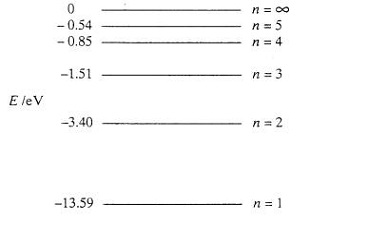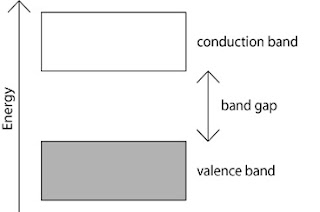## Recent

Theme images by Storman. Powered by Blogger.

# Energy Level and Energy Band

ENERGY LEVELS

The electrons nearer to the nucleus are more firmly held than those far away from the nucleus. The energy required to pull out the electron out the orbit will be more for the inner orbit than the outer orbit. Hence, the orbits are referred by the energy levels. The valency of an element is determined by the number of electrons it can receive or give away from its outermost orbit to another element in reaction. The element having 3 or less valence electrons give away their valence electrons but elements having 5 or more valence electrons will receive the balance electrons to make up the total of 8 for stability. The electrons in the outermost orbit are called valence electrons and are loosely bonded to the nucleus. A stable atom contains 8 valence electrons in the outermost orbit and an unstable atom contains less than 8 valence electrons.

Energy of Electron:

Consider the case of Hydrogen atom where the orbit is one and only one electron occupies it. The system will be stable when the electrostatic force between the nucleus and the electron is equal to the centrifugal force of the electron. As the orbit increases the energy level is inversely proportional to the square of orbit number. The closer an electron is to the nucleus, the stronger arc the forces that bind it. The energy level represents the amount of energy that would have to be applied to extract an electron from the orbit or shell.

An electron volt (eV) is defined as the amount of energy required to move one electron through a potential difference of one volt (1 eV = 1.60 x 10-19 J). The energy levels that may be occupied by electrons emerge as hands. There are two distinct bands within any given material in which electrons may exists. They are valence band and the conduction hand. The energy gap which separates these two bands is called forbidden gap within which no electrons can exists. Figure shows the same energy band diagram.Energy Level of an electron in Hydrogen AtomEnergy Band Diagram
When the number of electrons in the outermost orbit is more than four, the width of the forbidden gap is high and is classified as insulator. In the case of insulator, the amount of additional energy required for the electrons to escape the orbit has to be large so that the electrons can move to the conduction band. When the number of electrons in the outermost orbit is less than four, it would be easier to detach the electrons from the orbit with small amount of energy and is classified as conductor. Whereas in the case of semi-conductor the number of electrons in the outermost orbit is equal to four and thus it can tend to behave either as a conductor or insulator. In general, the resistivity of the material is directly related to the total energy required to detach the electron. If the energy required to detach the outermost electron is higher, the resistivity is also higher and vice-versa.